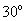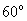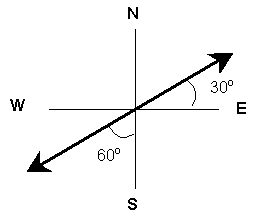Physics Department                 [Return to Examples]

# KINEMATICS  EXAMPLE   1A vector of magnitude 6m pointsnorth of east.  A second vector whose magnitude is 8m pointswest of south.  If we add these two vectors together, what is the resultant?Given:             Vector 1 magnitude = 6m, direction =north of east                        Vector 2 magnitude = 8m, direction =west of southUnknown:       Vector that results from adding these two, that is the magnitude and direction of the resultant vector.Physical (mathematical) Principles and Ideas:  rules for adding vectorsSolution:         Our sketch tells us that the two vectors lie on the same line, but point in opposite directions.  That means we will actually be subtracting one of these vectors from the other.  It is a little easier to subtract the smaller one from the larger, i.e., 8m – 6m = 2m.  Now we know the magnitude of our resultant.  By setting the subtraction up this way, what we have actually done is to make the 60o west of south (which is, of course, the same as 30o south of west) direction positive and the 30o north of east direction negative.  Since our value above is +2m, we know the direction is 60o west of south.  (What value would indicate a direction of 30o north of east if 60o west of south is positive?)All vector problems involving vectors that lie on a line are worked similarly.  Among the vector quantities we work with in physics are:  displacement, velocity, acceleration, force, momentum, and electric and magnetic fields.

Other Kinematics Examples:    1     2     3     4     5     6     7     8     9     10
[Examples Homepage]      [Physics Homepage]      [IPFW Homepage]